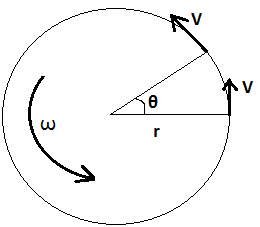#### A PHP Error was encountered

Severity: Warning

Message: count(): Parameter must be an array or an object that implements Countable

Filename: controllers/formulas.php

Line Number: 37

Angular Acceleration Formulas Online - Tutorpace

# Angular Acceleration Formula

## Online Tutoring Is The Easiest, Most Cost-Effective Way For Students To Get The Help They Need Whenever They Need It.

Angular acceleration of an object is the rate of change of angular velocity with respect to the time. It is similar to the linear acceleration but in a circular path.

It is denoted by alpha “α” and its S.I unit is radian/sec^2 or rad/s^2.
α = dω/dt
α = (ω2 – ω1)/(t2 – t1)ω = v/r
and, ω = θ/t
Where,       ω = angular velocity
t = time taken
v = linear velocity
r = radius of circular path
θ = rotated angle
ω1 and ω2 are the two different angular velocity at time t1 and t2 respectively.

Therefore,
α = d^2θ/dt^2

Example1: An object is rotating according to the function θ(t) = t3 + t, find the angular acceleration when t = 4 seconds?
Solution:   Given that,
Function of angular displacement, θ(t) = t3 + t
Time taken, t = 4 sec

Now, we have the equation for angular acceleration.
α = d^2θ/dt^2
= 6t = 6(4) = 24 rad/sec^2.

Example2: If an object changes it angular velocity from 20 rad/s to 50 rad/s in 6 seconds. Calculate angular acceleration?

Solution: Given that,